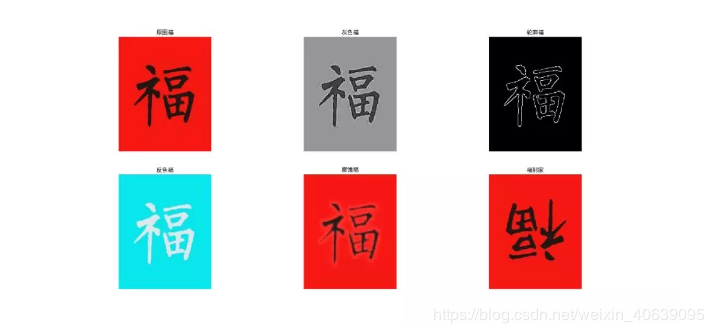# 新年福利来一波之Python轻松集齐五福(demo)``````
def Gray_fu(self):
gray_img = cv2.cvtColor(self.ori_img, cv2.COLOR_BGR2GRAY)
return gray_img``````

``````
def Canny_fn(self):
img = cv2.cvtColor(self.ori_img, cv2.COLOR_BGR2GRAY)
img = cv2.GaussianBlur(img, (3, 3), 0)
canny_img = cv2.Canny(img, 50, 150)
return canny_img``````

``````
def invert_fu(self):
img = 255 - self.ori_img
return img``````

``````
def dilate_fu(self):
# img = cv2.cvtColor(self.ori_img, cv2.COLOR_BGR2GRAY)
kernel = cv2.getStructuringElement(cv2.MORPH_RECT, (5, 5))
img = cv2.dilate(self.ori_img, kernel, iterations=1)
print(img.shape)
return img``````

``````
def rotate_fu(self):
img = cv2.rotate(self.ori_img, 1)
return img``````

``````
import cv2
class Change_pic():
def __init__(self, img):
def Gray_fu(self):
'''灰度图'''
gray_img = cv2.cvtColor(self.ori_img, cv2.COLOR_BGR2GRAY)
return gray_img
def Canny_fn(self):
'''轮廓图'''
img = cv2.cvtColor(self.ori_img, cv2.COLOR_BGR2GRAY)
img = cv2.GaussianBlur(img, (3, 3), 0)
canny_img = cv2.Canny(img, 50, 150)
return canny_img
def invert_fu(self):
'''反色图'''
img = 255 - self.ori_img
return img
def dilate_fu(self):
'''腐蚀图'''
# img = cv2.cvtColor(self.ori_img, cv2.COLOR_BGR2GRAY)
kernel = cv2.getStructuringElement(cv2.MORPH_RECT, (5, 5))
img = cv2.dilate(self.ori_img, kernel, iterations=1)
print(img.shape)
return img
def rotate_fu(self):
'''福到家'''
img = cv2.rotate(self.ori_img, 1)
return img``````

python 集齐五福 python集五福